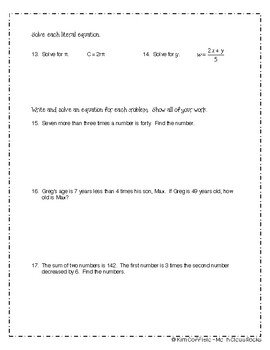# MULTI-STEP EQUATIONS REVIEW AND UNIT TESTSubject
Resource Type
Common Core Standards
Product Rating
File Type

PDF (Acrobat) Document File

1 MB|20 pages
Share
Product Description
This review and each unit test consists of 20 problems (plus one bonus problem) that focus on the following concepts:

1. One-Step Equations
2. Two-Step Equations
3. Combining Like Terms
4. Distributive Property
5. Variables on Both Sides
6. Literal Equations (transforming)
7. Write and solve a two-step equation
8. Write and solve an equation with like terms
9. Write and solve an equation with variables on both sides
10. Write and solve an equation with the distributive property

In addition to the review, there are two versions of the test. This works well when students are sitting in pairs or if you offer students the option to re-test.

Total Pages
20 pages